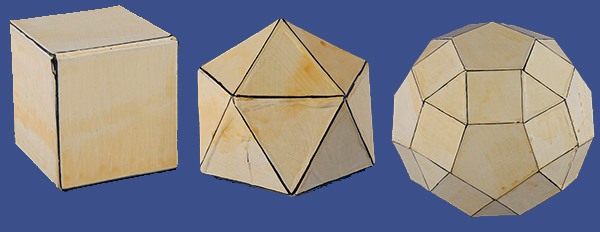# Geometric Models-Regular-Faced Convex Polyhedra -- Platonic Solids

## Platonic SolidsA polygon is regular if it is uniform and its faces are all alike. The regular convex polyhedra are the five Platonic solids, which have been known since classical Greece. The ancient Greek mathematician Euclid proved in his Elements of Geometry that there are only five Platonic solids – the regular tetrahedron (four sides that are equilateral triangles), the cube (six sides that are squares), the regular octahedron (eight sides that are equilateral triangles), the regular dodecahedron (twelve sides that are regular pentagons), and the regular icosahedron (twenty sides that are equilateral triangles).

## Contents